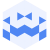# FELIX VIERLINGER | Tresor Linz I October 17 2023 | BestOFF Inside OutCreated at 13. Nov. 2023

76 Ansichten
##### by Tresor Linz

Tresor Linz - recorded on October 17 2023

<<<<<<<<<<<<<<<<<<<<<<<<<<<<<<<<<<<<<<<<<<<<<<<<<<<<<<<<<
FELIX VIERLINGER - Scratching matter N01
<<<<<<<<<<<<<<<<<<<<<<<<<<<<<<<<<<<<<<<<<<<<<<<<<<<<<<<<<
(Postdigital Lutherie)

de:
Repetitive Abläufe kreieren Wirklichkeiten und schaffen Bezüge. Immer Wiederkehrendes scheint bekannt zu
sein. Aus Rhythmusstrukturen, die für die Performance aus unzähligen Endlosrillen gestaltet wurden, wird durch Überlagerungen ein akustischer Teppich geknüpft. Strukturen vernetzen sich zu einem fragilen Verbund. SCRATCHING MATTER ist eine Performance für fünf Plattenspieler und eigens geritzte Endlosrillen.

en:
Repetitive sequences create realities and establish references. The recurring seems to be familiar. Designed for performance with countless endless grooves, rhythm structures are woven into an acoustic tapestry through overlays. Structures interlink to forma fragile compound. SCRATCHING MATTER is a performance for five turntables and specially scratched endless grooves.

<<<<<<<<<<<<<<<<<<<<<<<<<<<<<<<<<<<<<<<<<<<<<<<<<<<<<<<<<
Tresor Linz
<<<<<<<<<<<<<<<<<<<<<<<<<<<<<<<<<<<<<<<<<<<<<<<<<<<<<<<<<

Raum für Klangkunst und künstlerische Experimente.

Space for sound art and artistic experiments.

##### Share & Embed
Embed this Video